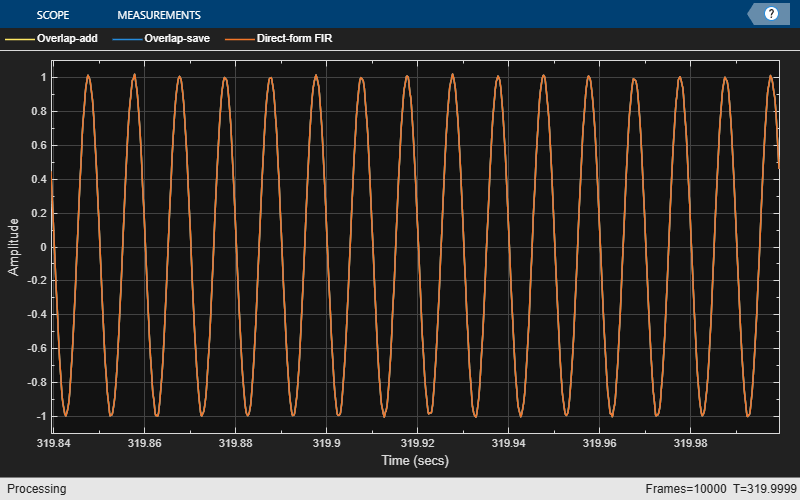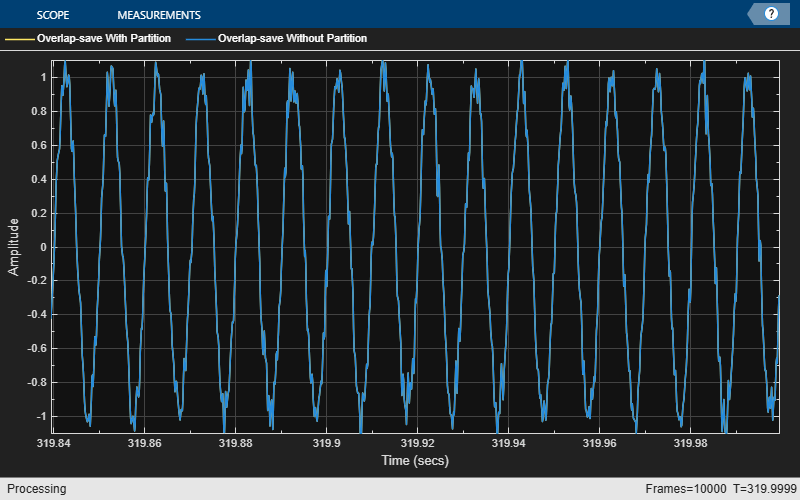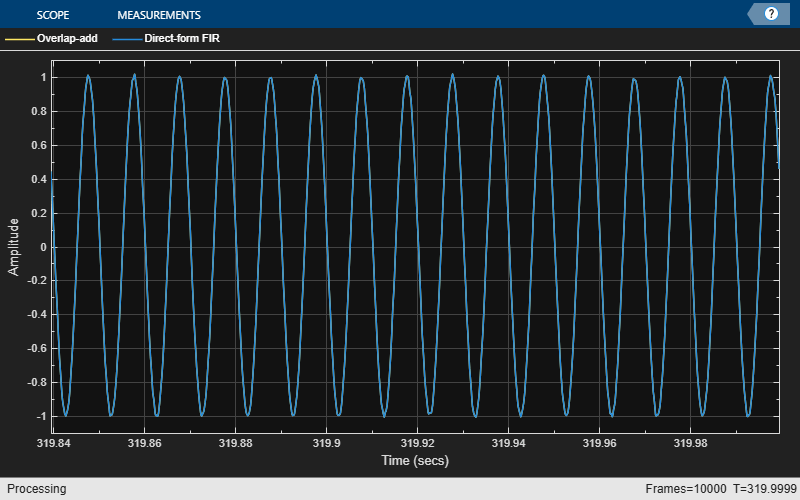Documentation

# dsp.FrequencyDomainFIRFilter

Filter input signal in frequency domain

## Description

The `dsp.FrequencyDomainFIRFilter` System object™ implements frequency-domain, fast Fourier transform (FFT)-based filtering to filter a streaming input signal. In the time domain, the filtering operation involves a convolution between the input and the impulse response of the finite impulse response (FIR) filter. In the frequency domain, the filtering operation involves the multiplication of the Fourier transform of the input and the Fourier transform of the impulse response. The frequency-domain filtering is efficient when the impulse response is very long. You can specify the filter coefficients directly in the frequency domain by setting `NumeratorDomain` to `'Frequency'`.

This object uses the overlap-save and overlap-add methods to perform the frequency-domain filtering. For filters with a long impulse response length, the latency inherent to these two methods can be significant. To mitigate this latency, the `dsp.FrequencyDomainFIRFilter` object partitions the impulse response into shorter blocks and implements the overlap-save and overlap-add methods on these shorter blocks. To partition the impulse response, set the PartitionForReducedLatency property to `true`. For more details on these two methods and on reducing latency through impulse response partitioning, see Algorithms.

To filter the input signal in the frequency domain:

1. Create the `dsp.FrequencyDomainFIRFilter` object and set its properties.

2. Call the object with arguments, as if it were a function.

To learn more about how System objects work, see What Are System Objects? (MATLAB).

## Creation

### Syntax

``fdf = dsp.FrequencyDomainFIRFilter``
``fdf = dsp.FrequencyDomainFIRFilter(num)``
``fdf = dsp.FrequencyDomainFIRFilter(Name,Value)``

### Description

````fdf = dsp.FrequencyDomainFIRFilter` creates a frequency domain FIR filter System object that filters each channel of the input signal independently over time in the frequency domain using the overlap-save or overlap-add method.```

example

````fdf = dsp.FrequencyDomainFIRFilter(num)` creates a frequency domain FIR filter object with the Numerator property set to `num`.Example: ```dsp.FrequencyDomainFIRFilter(fir1(400,2 * 2000 / 8000));``````

example

````fdf = dsp.FrequencyDomainFIRFilter(Name,Value)` creates a frequency domain FIR filter System object with each specified property set to the specified value. Enclose each property name in single quotes. You can use this syntax with any previous input argument combinations.Example: `dsp.FrequencyDomainFIRFilter('Method','Overlap-add');````

## Properties

expand all

Unless otherwise indicated, properties are nontunable, which means you cannot change their values after calling the object. Objects lock when you call them, and the `release` function unlocks them.

If a property is tunable, you can change its value at any time.

For more information on changing property values, see System Design in MATLAB Using System Objects (MATLAB).

Frequency-domain filter method, specified as either `'Overlap-save'` or `'Overlap-add'`. For more details on these two methods, see Algorithms.

Domain of the filter coefficients, specified as one of the following:

• `'Time'` –– Specify the time-domain filter numerator in the `Numerator` property.

• `'Frequency'` –– Specify the filter's frequency response in the `FrequencyResponse` property.

FIR filter coefficients, specified as a row vector.

Tunable: Yes

#### Dependencies

This property applies when `NumeratorDomain` is set to `'Time'`.

Data Types: `single` | `double` | `int8` | `int16` | `int32` | `int64` | `uint8` | `uint16` | `uint32` | `uint64`
Complex Number Support: Yes

Frequency response of the filter, specified as a row vector or a matrix. When `PartitionForReducedLatency` is `false`, `FrequencyResponse` must be a row vector. The FFT length is equal to the length of the `FrequencyResponse` vector. When `PartitionForReducedLatency` is `true`, `FrequencyResponse` must be a 2P-by-N matrix, where P is the partition size, and N is the number of partitions.

Tunable: Yes

#### Dependencies

This property applies when `NumeratorDomain` is set to `'Frequency'`.

Data Types: `single` | `double` | `int8` | `int16` | `int32` | `int64` | `uint8` | `uint16` | `uint32` | `uint64`
Complex Number Support: Yes

Time-domain numerator length, specified as a positive integer-valued scalar.

#### Dependencies

This property applies when `NumeratorDomain` is set to `'Frequency'` and `PartitionForReducedLatency` is set to `false`.

Data Types: `single` | `double` | `int8` | `int16` | `int32` | `int64` | `uint8` | `uint16` | `uint32` | `uint64`

Flag to specify if the filter coefficients are all real, specified as `true` or `false`.

#### Dependencies

This property applies when `NumeratorDomain` is set to `'Frequency'`.

Flag to partition numerator to reduce latency, specified as one of the following:

• `false` –– The filter uses the traditional overlap-save or overlap-add method. The latency in this case is FFTLength – length(Numerator) + 1.

• `true` –– In this mode, the object partitions the numerator into segments of length specified by the PartitionLength property. The filter performs overlap-save or overlap-add on each partition, and combines the partial results to form the overall output. The latency is now reduced to the partition length.

FFT length, specified as a positive integer. The default value of this property, `[]`, indicates that the FFT length is equal to twice the numerator length. The FFT length must be greater than or equal to the numerator length.

Example: `64`

#### Dependencies

This property applies when you set `NumeratorDomain` property to `'Time'` and PartitionForReducedLatency property to `false`.

Data Types: `single` | `double` | `int8` | `int16` | `int32` | `int64` | `uint8` | `uint16` | `uint32` | `uint64`

Numerator partition length, specified as a positive integer less than or equal to the length of the numerator.

Example: `40`

Example: `60`

#### Dependencies

This property applies when you set the `NumeratorDomain` property to `'Time'` and PartitionForReducedLatency property to `true`.

Data Types: `single` | `double` | `int8` | `int16` | `int32` | `int64` | `uint8` | `uint16` | `uint32` | `uint64`

This property is read-only.

Filter latency in samples, returned as an integer greater than 0. When PartitionForReducedLatency is `false`, the latency is equal to FFTLength – length(Numerator) + 1. When `PartitionForReducedLatency` is `true`, the latency is equal to the partition length.

Data Types: `uint32`

## Usage

### Syntax

``fdfOut = fdf(input)``

### Description

example

````fdfOut = fdf(input)` filters the input signal and outputs the filtered signal. The object filters each channel of the input signal independently over time in the frequency domain.```

### Input Arguments

expand all

Data input, specified as a vector or a matrix. This object supports variable-size input signals. That is, you can change the input frame size (number of rows) even after calling the algorithm. However, the number of channels (number of columns) must remain constant.

Example: `randn(164,4)`

Data Types: `single` | `double`
Complex Number Support: Yes

### Output Arguments

expand all

Filtered output, returned as a vector or matrix. The size, data type, and complexity of the output match those of the input.

Data Types: `single` | `double`
Complex Number Support: Yes

## Object Functions

To use an object function, specify the System object as the first input argument. For example, to release system resources of a System object named `obj`, use this syntax:

`release(obj)`

expand all

 `fvtool` Visualize frequency response of DSP filters
 `step` Run System object algorithm `release` Release resources and allow changes to System object property values and input characteristics `reset` Reset internal states of System object

## Examples

expand all

Filter input signal using overlap-add and overlap-save methods, and compare the outputs to the output of a FIR filter.

Initialization

Design the FIR lowpass filter coefficients using the `fir1` function. The sampling frequency is 8 kHz, and the cutoff frequency of the filter is 2 kHz. The impulse response has a length of 400.

```impL = 400; Fs = 8000; Fcutoff = 2000; imp = fir1(impL,2*Fcutoff/Fs); ```

Create two `dsp.FrequencyDomainFIRFilter` objects and a `dsp.FIRFilter` object. Set the numerator of all the three filters to `imp`. Delay the FIR output by the latency of the frequency-domain filter.

```fdfOA = dsp.FrequencyDomainFIRFilter(imp,'Method','overlap-add'); fdfOS = dsp.FrequencyDomainFIRFilter(imp,'Method','overlap-save'); fir = dsp.FIRFilter('Numerator',imp); dly = dsp.Delay('Length',fdfOA.Latency); ```

Create two `dsp.SineWave` objects. The sine waves generated have a sample rate of 8000 Hz, frame size of 256, and frequencies of 100 Hz and 3 kHz, respectively. Create a `dsp.TimeScope` object to view the filtered outputs.

```frameLen = 256; sin_100Hz = dsp.SineWave('Frequency',100,'SampleRate',Fs,... 'SamplesPerFrame',frameLen); sin_3KHz = dsp.SineWave('Frequency',3e3,'SampleRate',Fs,... 'SamplesPerFrame',frameLen); ts = dsp.TimeScope('TimeSpanOverrunAction','Scroll',... 'ShowGrid',true,'TimeSpan',5 * frameLen/Fs,... 'YLimits',[-1.1 1.1],... 'ShowLegend',true,... 'SampleRate',Fs,... 'ChannelNames',{'Overlap-add','Overlap-save','Direct-form FIR'}); ```

Streaming

Stream 1e4 frames of noisy input data. Pass this data through the frequency domain filters and the FIR filter. View the filtered outputs in the time scope.

```numFrames = 1e4; for idx = 1:numFrames x = sin_100Hz() + sin_3KHz() + 0.01*randn(frameLen,1); yOA = fdfOA(x); yOS = fdfOS(x); yFIR = fir(dly(x)); ts([yOA,yOS,yFIR]); end ```The outputs of all the three filters match exactly.

Partition the impulse response length of a frequency domain FIR filter. Compare the outputs of the partitioned filter and the original filter.

Design the FIR lowpass filter coefficients using the `fir1` function. The sampling frequency is 8 kHz and the cutoff frequency of the filter is 2 kHz. The impulse response is of length 4000.

```impL = 4000; Fs = 8000; Fcutoff = 2000; imp = fir1(impL,2 * Fcutoff / Fs); ```

Create a `dsp.FrequencyDomainFIRFilter` with coefficients set to the `imp` vector. The latency of this filter is given by, which is equal to 4002. By default, FFT length is equal to twice the numerator length. This makes the latency proportional to the impulse response length.

```fdfOS = dsp.FrequencyDomainFIRFilter(imp,'Method','overlap-save'); fprintf('Frequency domain filter latency is %d samples\n',fdfOS.Latency); ```
```Frequency domain filter latency is 4002 samples ```

Partition the impulse response to blocks of length 256. The latency after partitioning is proportional to the block length.

```fdfRL = dsp.FrequencyDomainFIRFilter(imp,'Method','overlap-save',... 'PartitionForReducedLatency',true,... 'PartitionLength',256); fprintf('Frequency domain filter latency is %d samples\n',fdfRL.Latency); ```
```Frequency domain filter latency is 256 samples ```

Compare the outputs of the two frequency domain filters. The latency of `fdfOS` is 4002, and the latency of `fdfRL` is 256. To compare the two outputs, delay the input to `fdfRL` by 4002 - 256 samples.

```dly = dsp.Delay('Length',(fdfOS.Latency-fdfRL.Latency)); ```

Create two `dsp.SineWave` objects. The sine waves have a sample rate of 8000 Hz, frame size of 256, and frequencies of 100 Hz and 3 kHz, respectively. Create a `dsp.TimeScope` object to view the filtered outputs.

```frameLen = 256; sin_100Hz = dsp.SineWave('Frequency',100,'SampleRate',Fs,... 'SamplesPerFrame',frameLen); sin_3KHz = dsp.SineWave('Frequency',3e3,'SampleRate',Fs,... 'SamplesPerFrame',frameLen); ts = dsp.TimeScope('TimeSpanOverrunAction','Scroll',... 'ShowGrid',true,'TimeSpan',5 * frameLen/Fs,... 'YLimits',[-1.1 1.1],... 'ShowLegend',true,... 'SampleRate',Fs,... 'ChannelNames',{'Overlap-save With Partition','Overlap-save Without Partition'}); ```

Stream 1e4 frames of noisy input data. Pass this data through the two frequency domain filters. View the filtered outputs in the time scope.

```numFrames = 1e4; for idx = 1:numFrames x = sin_100Hz() + sin_3KHz() + .1 * randn(frameLen,1); yRL = fdfRL(dly(x)); yOS = fdfOS(x); ts([yRL,yOS]); end ```The outputs match exactly.

Specify the numerator coefficients of the frequency-domain FIR filter in the frequency domain. Filter the input signal using the overlap-add method. Compare the frequency-domain FIR filter output to the corresponding time-domain FIR filter output.

Initialization

Design the FIR lowpass filter coefficients using the `fir1` function. The sampling frequency is 8 kHz, and the cutoff frequency of the filter is 2 kHz. The time-domain impulse response has a length of 400. Compute the FFT of this impulse response and specify this response as the frequency response of the frequency-domain FIR filter. Set the time-domain numerator length, specified by the `NumeratorLength` property, to the number of elements in the time-domain impulse response.

```impL = 400; Fs = 8000; Fcutoff = 2000; imp = fir1(impL,2 * Fcutoff / Fs); H = fft(imp , 2 * numel(imp)); oa = dsp.FrequencyDomainFIRFilter('NumeratorDomain','Frequency',... 'FrequencyResponse', H,... 'NumeratorLength',numel(imp),... 'Method','overlap-add'); fprintf('Frequency domain filter latency is %d samples\n',oa.Latency); ```
```Frequency domain filter latency is 402 samples ```

Create a `dsp.FIRFilter` System object? and specify the numerator as the time-domain coefficients computed using the `fir1` function, `imp`. Delay the FIR output by the latency of the frequency-domain FIR filter.

```fir = dsp.FIRFilter('Numerator',imp); dly = dsp.Delay('Length',oa.Latency); ```

Create two `dsp.SineWave` objects. The sine waves generated have a sample rate of 8000 Hz, frame size of 256, and frequencies of 100 Hz and 3 kHz, respectively. Create a `dsp.TimeScope` object to view the filtered outputs.

```frameLen = 256; sin_100Hz = dsp.SineWave('Frequency',100,'SampleRate',Fs,... 'SamplesPerFrame',frameLen); sin_3KHz = dsp.SineWave('Frequency',3e3,'SampleRate',Fs,... 'SamplesPerFrame',frameLen); ts = dsp.TimeScope('TimeSpanOverrunAction','Scroll',... 'ShowGrid',true,'TimeSpan',5 * frameLen/Fs,... 'YLimits',[-1.1 1.1],... 'ShowLegend',true,... 'SampleRate',Fs,... 'ChannelNames',{'Overlap-add','Direct-form FIR'}); ```

Streaming

Stream 1e4 frames of noisy input data. Pass this data through the frequency-domain FIR filter and the time-domain FIR filter. View the filtered outputs in the time scope.

```numFrames = 1e4; for idx = 1:numFrames x = sin_100Hz() + sin_3KHz() + 0.01 * randn(frameLen,1); y1 = oa(x); y2 = fir(dly(x)); ts([y1,y2]); end ```The outputs of both the filters match exactly.

## Algorithms

expand all

Overlap-save and overlap-add are the two frequency-domain FFT-based filtering methods this algorithm uses.

 Stockham, T. G., Jr. "High Speed Convolution and Correlation." Proceedings of the 1966 Spring Joint Computer Conference, AFIPS, Vol 28, 1966, pp. 229–233.

Watch now